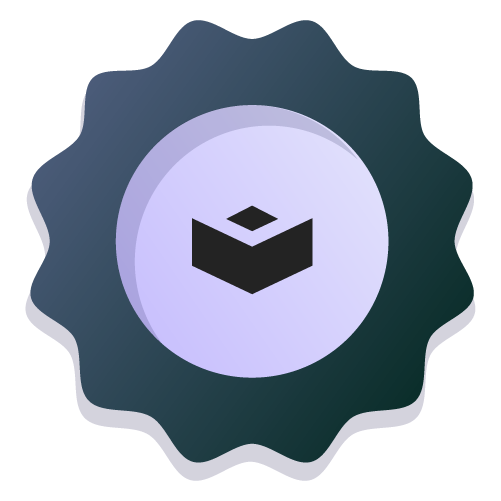Classification in Depth with Scikit-Learn

# Logistic Regression

During this project, you'll learn about Logistic Regression using a real dataset. The objective is to diagnose whether or not a patient has diabetes based on diagnostic measurements included in the dataset. Using this example, you will learn the foundation of logistic regression, how to implement it using scikit-learn, and understand the decision boundaries of this model.

## Project Activities

All our Data Science projects include bite-sized activities to test your knowledge and practice in an environment with constant feedback.

All our activities include solutions with explanations on how they work and why we chose them.

codevalidated

### Logistic Function

Now, create a set of x-values, using np.linspace() function, with a range from -10 to 10 and 100 data points. Store the result in the variable `x`.

Next, we use the logistic function to calculate \$y\$ values (Store the result in the variable `y`), and finally, we use matplotlib.pyplot to plot the \$x\$,\$y\$ values.

The resulting plot will show the S-shaped curve of the logistic function, which is useful for modeling probabilities.

multiplechoice

codevalidated

### Standardize the data

Use `StandardScaler` to standardize the features and store the results in the variables `X_train_scaler` and `X_test_scaler`.

codevalidated

### Use the model to make predictions on the test data and evaluate its accuracy score, based on the following code:

``````y_pred = logreg.predict(---)
print("Accuracy:", logreg.score(---, ---))
``````
input

### Fit a logistic regression model using the given input and target values. Once fitted, obtain the first coeficient of this model.

``````from sklearn.datasets import make_blobs

X, y = make_blobs(n_samples=1000, centers=2,
random_state=0, cluster_std=0.85)
``````

For the model set random_state = 0.

Round to three decimal places

input

### Fit a logistic regression model using the given input and output patterns X and Y, respectively. Once fitted, determine the accuracy score of the test dataset

``````from sklearn.datasets import make_blobs

X, y = make_blobs(n_samples=1000, centers=2,
random_state=49, cluster_std=1.95)
``````

For the model set random_state = 0.

Test dataset:

``````X_test= [[0.77499332, 5.10445441],
[2.33615249, 3.733497  ],
[3.53929109, 1.13492994],
[2.82894249, 4.00864077],
[4.61800816, 2.39809546]]

y_test=[0, 1, 1, 0, 0]
``````

Round to two decimal placesAuthor

#### Verónica Barraza

This project is part of

## Classification in Depth with Scikit-Learn

Explore other projectsAnurag VermaAnurag VermaSantiago Basulto